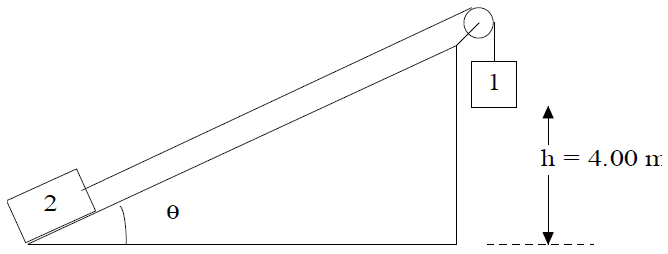# Finding speed using energy equations

## Homework Statement

Two masses are connected by a light string running over a frictionless pulley as shown below. The system is initially at rest and the incline is frictionless. If m1 = 10.0 kg, m2 = 8.00 kg and the incline makes a 30o angle from the horizontal, use energy methods to find the speed of the 8.00 kg mass just as the 10.0 kg mass reaches the ground 4.00 m below. (Hint: Think about how far the second mass will rise vertically as the first mass drops 4.00 m downward.)## Homework Equations

KEf + Uf = KEi + Ui
(.5)mvf² + mgyf = (.5)mvi² + mgyi

## The Attempt at a Solution

I'm really not sure what to do here. Here is one of my attempts.

(.5)(18)(vf)² +(18)(9.8)(0) = (.5)(18)(0)² + (18)(9.8)(4)

Solving for vf I get vf = 8.854, which is not the correct answer.

I'm pretty confused here and am not even sure if I am using the equation I need to be using. Any help would be greatly appreciated.

Thanks

PhanthomJay
Homework Helper
Gold Member

## Homework Statement

Two masses are connected by a light string running over a frictionless pulley as shown below. The system is initially at rest and the incline is frictionless. If m1 = 10.0 kg, m2 = 8.00 kg and the incline makes a 30o angle from the horizontal, use energy methods to find the speed of the 8.00 kg mass just as the 10.0 kg mass reaches the ground 4.00 m below. (Hint: Think about how far the second mass will rise vertically as the first mass drops 4.00 m downward.)

## Homework Equations

KEf + Uf = KEi + Ui
(.5)mvf² + mgyf = (.5)mvi² + mgyi

## The Attempt at a Solution

I'm really not sure what to do here. Here is one of my attempts.

(.5)(18)(vf)² +(18)(9.8)(0) = (.5)(18)(0)² + (18)(9.8)(4)

Solving for vf I get vf = 8.854, which is not the correct answer.

I'm pretty confused here and am not even sure if I am using the equation I need to be using. Any help would be greatly appreciated.

Thanks
You essentially have the right equation, but you are not correctly calculating the potential energy U correctly. For example, initially, one mass is 4 m above ground, and the other is at the ground. They have different potential energies, both initially and finaly. They do, however, have the same speed, so your Kinetic energy terms are correct.

Ok I got that Yf of mass2 is 2. And if I write the equation for each mass I get:

mass1
(.5)(18)(vf)² + (18)(9.8)(0) = (.5)(18)(0) + (18)(9.8)(4)

mass2
(.5)(18)(vf)² + (18)(9.8)(2) = (.5)(18)(0) + (18)(9.8)(0)

Is that right? Does that help me at all?

PhanthomJay